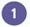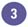# Displaying Calculations with AutoCalculate

You can simplify your work using a feature called AutoCalculate when you don't want to insert a formula, but you want to see the results of a simple calculation quickly. Auto-Calculate automatically displays the sum, average, maximum, minimum, or count of the selected values on the status bar. Auto-Calculate results do not appear on the worksheet when printed but are useful for giving you quick answers while you work.

#### Calculate a Range AutomaticallySelect the range of cells you want to calculate.

The sum of the selected cells appears on the status bar next to SUM=.If you want to change the type of calculation AutoCalculate performs , right-click anywhere on the status bar to open the AutoCalculate sub menu.Click the type of calculation you want.Show Me Microsoft Office Excel 2003
ISBN: 0789730057
EAN: 2147483647
Year: 2002
Pages: 291